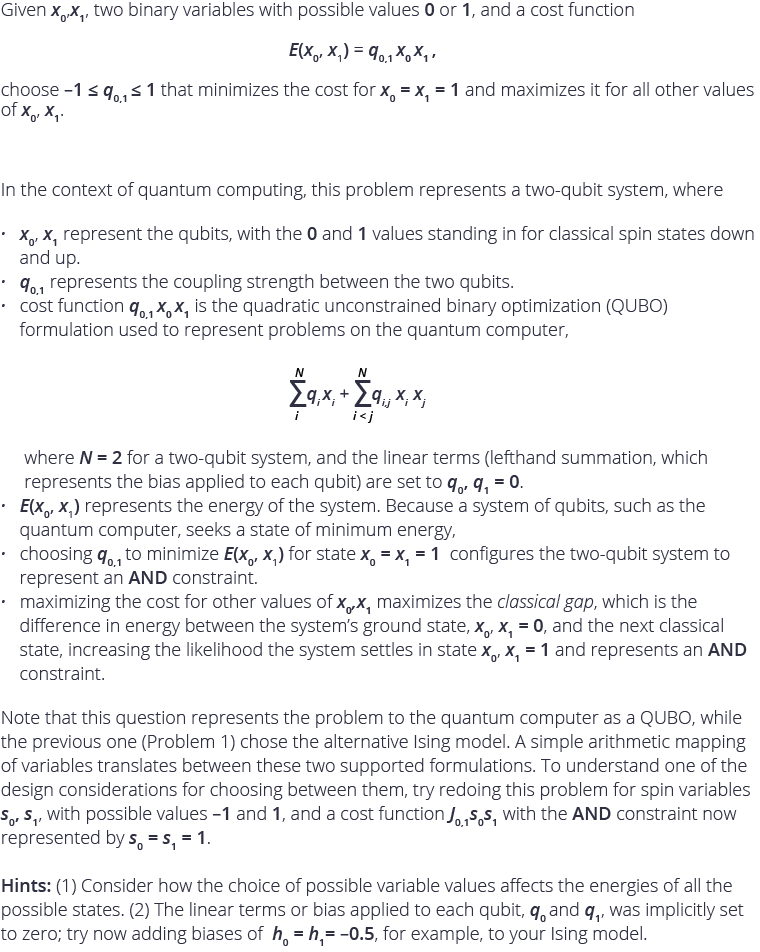# Problem 2: Represent an AND Constraint on a Two-Qubit System• hm, looking at the formula, i think the Q must be equal -1 to minimize the cost function? :-)

• `dimod.BinaryQuadraticModel({1: 0, 2: 0},{(1, 2): Q}, 0.0, dimod.BINARY) will be AND a gate when Q=-1.  `
•• The answer to Problem 2 is -1.

Great answers from Branislav, Yasas, and Jacob. Congratulations to Branislav for being first.

Check out Problem 3!